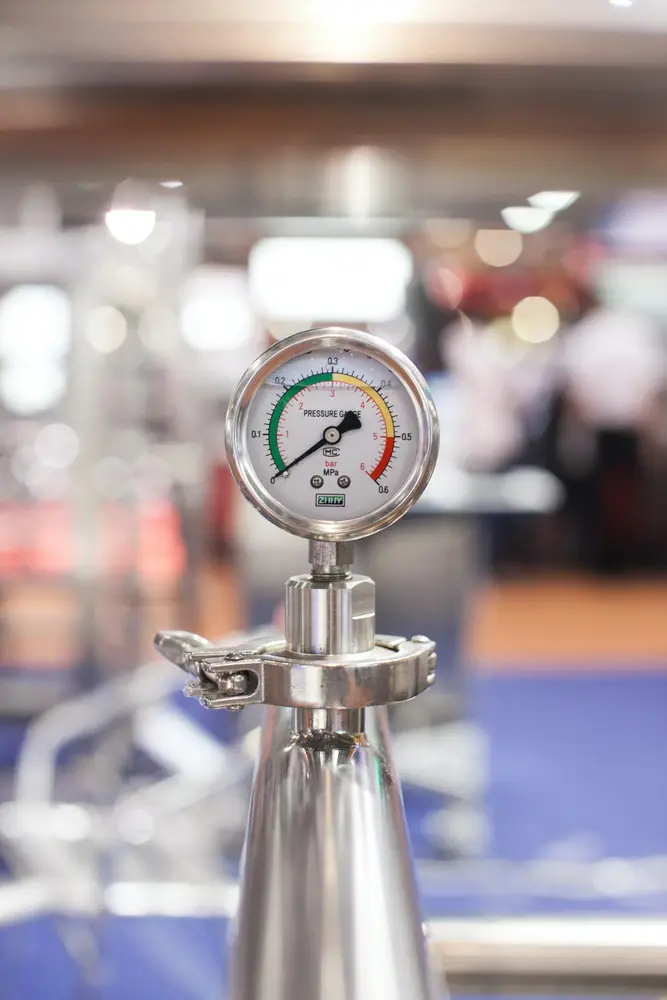# Momentum

• Momentum is the measure of how difficult it is to change an objects' velocity.
• Momentum can be increased by increasing the mass of the object or the velocity of the object.
• e.g. a lorry going at 80km/h down a hill with a very heavy load would have a big momentum. - It would be very difficult to stop! (If this was an exam question you would have to convert hours to seconds and km to m)
• The formula for calculating momentum is:
• Momentum (kgm/s) = mass (kg) x velocity (m/s)
• Conservation of momentum just shows that momentum is never lost but just transferred from one object to another e.g. if the big lorry runs into a car then all the momentum will be transferred to the car.
Forces and motion

#### Acceleration

##### Acceleration

Forces and motion

#### Forces

##### Forces

Forces and motion

#### Forumulae

##### Forumulae

Forces and motion

#### Hooke's Law

##### Hooke's Law

Forces and motion

#### Pressure

##### Pressure

Forces and motion